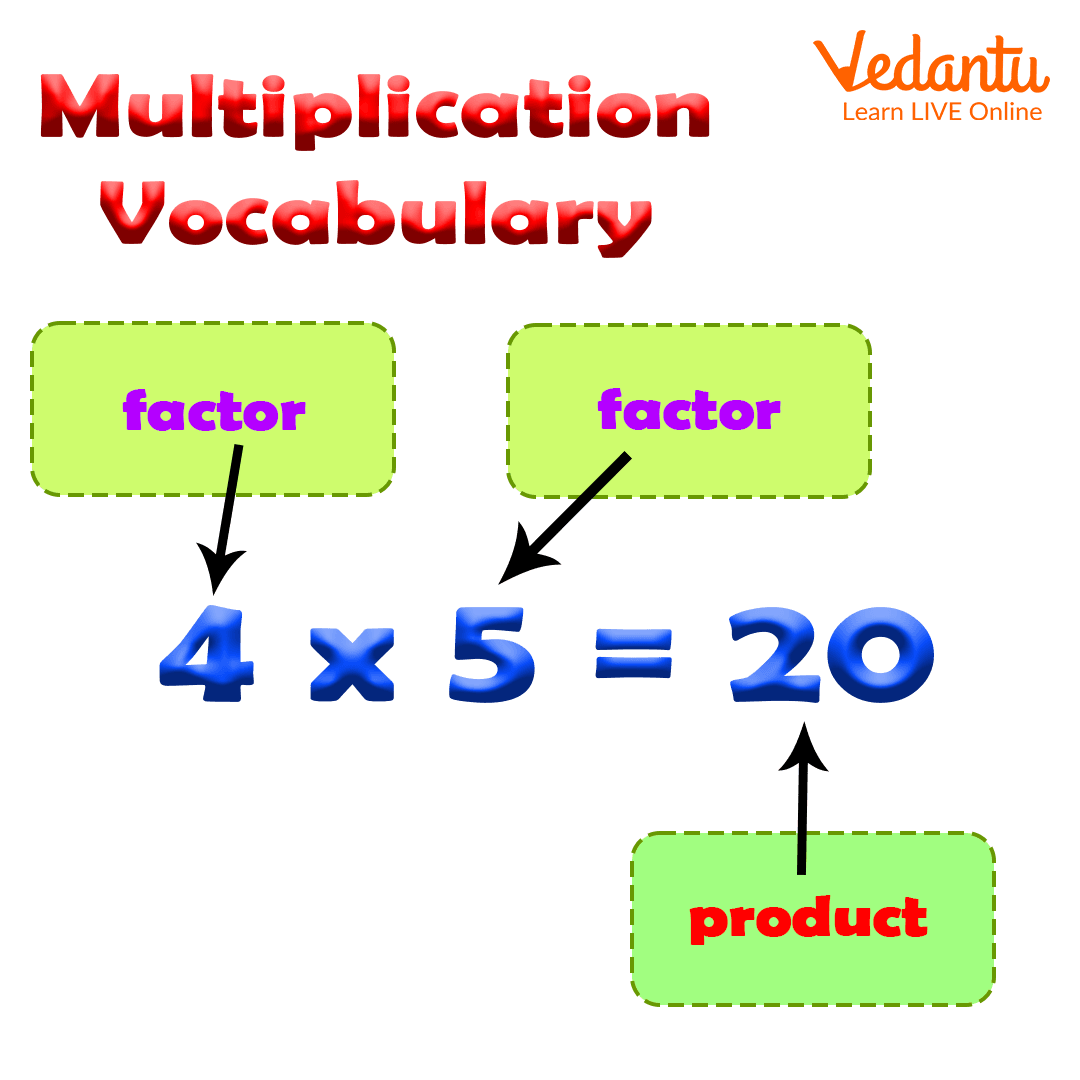Courses
Courses for Kids
Free study material
Free LIVE classes
More

# List of Multiplication Vocabulary and Properties of MultiplicationLIVE
Join Vedantu’s FREE Mastercalss

## An Introduction to Multiplication Vocabulary

There are four basic operations of Mathematics; these are addition, subtraction, multiplication, and division. So, multiplication is one of the elementary operations of computation in Mathematics. There are a lot of terminologies that are used in multiplication which come under multiplication vocabulary. The multiplication of two numbers (e.g. 2 2) is equivalent to adding as many copies of one of them. We are required to know the meaning of these terminologies. In this article, we will learn about the words of multiplication vocabulary and their meaning as well.Basic terminology of multiplication

### The List of Multiplication Vocabulary words

The list of multiplication vocabulary words is given below with their meanings.

 Sr. No. Words Meaning 1 Multiple Multiple is defined as the product of a given whole number with any whole number. 2 Factor Numbers that are used in multiplication for multiplying together to get a product. 3 Multiplier Multiplier is the number with which we perform the operation of multiplication. 4 Multiplicand In the process of multiplication, the number to be multiplied is termed the multiplicand. 5 Product Product is the result we obtain when we multiply two factors together. 6 Square number The square number is the product of a number multiplied by itself. For example, 22=4. 7 Composite number A composite number is a whole number that is greater than one that has more than two different factors. 8 Multiplication The multiplication of two numbers is similar to doing the addition of many copies of one of them.

### Properties of Multiplication

There are some important properties that are related to multiplication which are discussed below.

1. Commutative property of multiplication

According to the commutative property of multiplication, when the order of multiplier and multiplicand are changed, the product does not change.

For example, 8 × 6 = 48 or 6 × 8 = 48

So, 8 × 6 = 6 × 8.

1. Associative property of multiplication

According to the associative property of multiplication, the product of three or more numbers does not change if we change the grouping of the numbers.

For example: (3 × 8) × 5 = 24 × 5 = 120

or, (3 × 5) × 8 = 15 × 8 = 120

or, (8 × 5) × 3 = 40 × 3 = 120

1. One property of multiplication

According to one property of multiplication, the product of a number and 1 is the number itself.

For example:

4 × 1 = 4

5 × 1 = 5

23 × 1 = 23

21× 1 = 21

1. Zero property of multiplication

According to the zero property of multiplication, the result of multiplication of any number and zero is zero.

For example:

6 × 0 = 0

0 × 34 = 0

8 × 0 = 0

80 × 0 = 0

1. Distributive property of multiplication

According to the distributive property of multiplication, the product of a number and the sum of two numbers is always the same as the sum of the product of the numbers.

For example:

6 × (3 + 8) = 6 × 11 = 66

6 × 3 + 6 × 8 = 66

So, 6 × (3 + 8) = 6 × 3 + 6 × 8 = 66

In the same way, the product of a number and the difference of two numbers is also always the same as the difference of the product of the numbers.

For example:

3 × (6 − 5) = 3

3 × 6 − 3 × 5 = 18 − 15 = 3

So, 3 × (6 − 5) = 3 × 6 − 3 × 5 = 3

### Conclusion

In the above article, all the words related to multiplication are described and the properties of multiplication are explained with examples. This article will help students to develop a strong concept of multiplication.

Last updated date: 16th Sep 2023
Total views: 136.5k
Views today: 1.36k

## FAQs on List of Multiplication Vocabulary and Properties of Multiplication

1. What do you understand by multiplication?

Multiplication is one of the four elementary Mathematical operations of arithmetic calculations. Hence, it is an important operation of Mathematical computation. The result of the operation of multiplication is termed as product. The multiplication of whole numbers can be understood by considering repeated addition because the multiplication of two numbers is equivalent to adding as many copies of one of them.

2. What are the vocabulary words for multiplication?

There are different terminologies in multiplication such as factor, multiplier, multiplicand, product, etc. These are the basic words used in terminology. A multiplier is the digits or number by which a multiplicand is multiplied while the multiplicand is defined as the number that is multiplied by the multiplier. A product is the result of the multiplication.